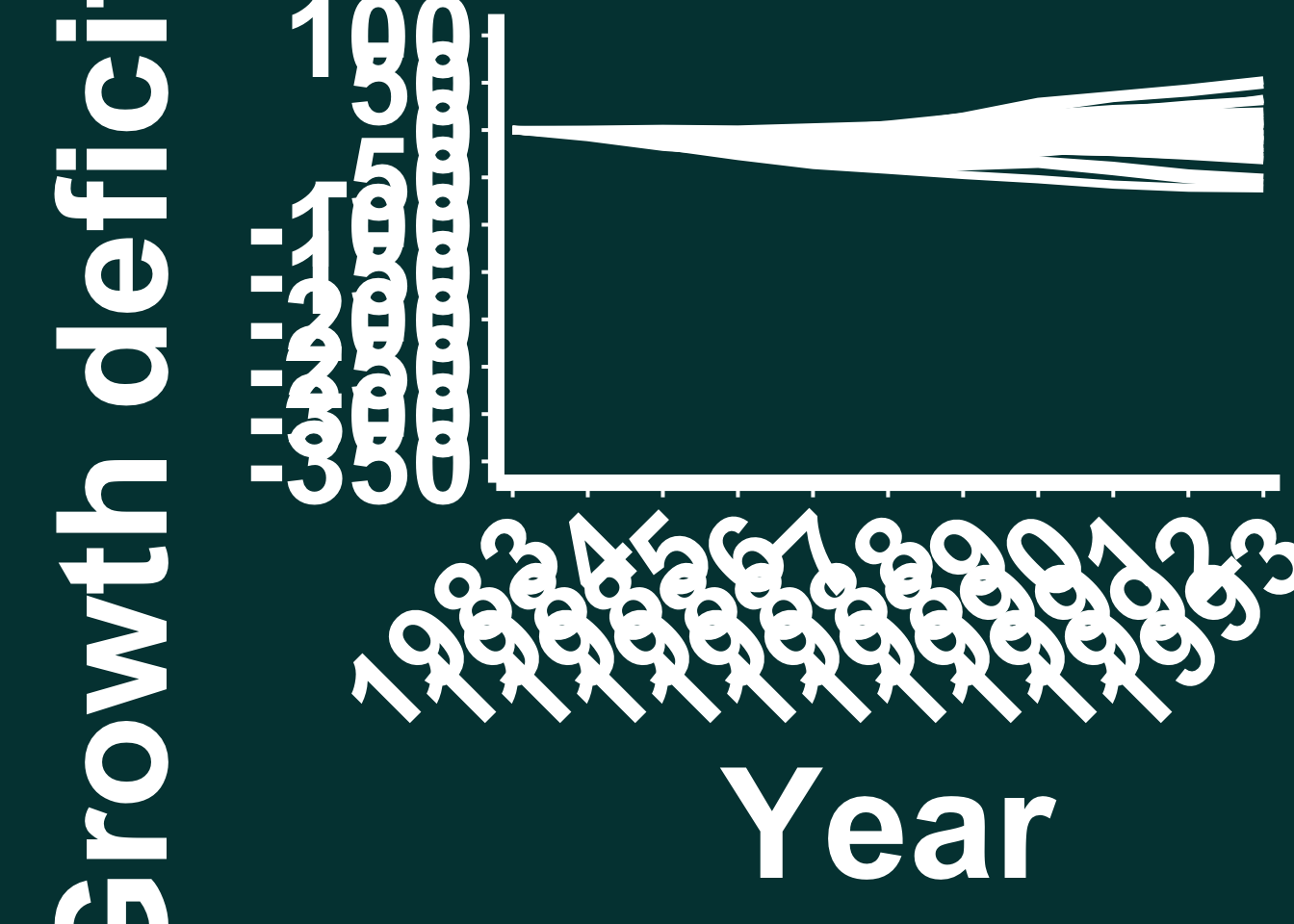Comments: Load in ggplot (install it if you don’t already have it) and then set your working directory to where you have saved the downloaded data by changing the below file path. Then, read in the data using the .csv command.

library(ggplot2)

setwd("//Users/macbookpro/Creative Cloud Files/YouTube SciComm Tutorial Series/3. Basic animation - animating a graph in After Effects/R_Data/")

AE_Example_data <- read.csv("FR6_BA_BAI_Forecast_vs_Actual.csv")

Comments: Once you have loaded in the ggplot package, set your working directory and loaded in the data using the above steps, we can generate a figure from this data using ggplot code below. Here we store this plot as an object called “AE_Plot” which we subsequently call (don’t worry that it looks badly formatted, we have set the axis and text sizing intentionally so it exports correctly - it just looks a mess when we call the ggplot in R!), then re-set our working directory to wherever we want to save a .png image of our plot and then use ggsave to save this ggplot as a .png file.

NOTE - As detailed in the tutorial, here we have intentionally saved the ggplot here as a .png file is to demonstrate how the colours of a PNG do not match the colours used in After Effects, despite using the same HEX colour codes in the ggplot code (in our example here, we use HEX colour #004040). We then use this PNG in this tutorial to show how we can use illustrator to get around this problem if all we have is a PNG and don’t have the ability to save a graph as an EPS file. Normally, when saving a plot for use in After Effects to ensure the colours are the same, be sure to save your figure via the “Export” button in the “Plots” panel and save it as an “EPS” not a “PNG” file.

AE_Plot <- ggplot() +
geom_line(data = AE_Example_data, aes(x = Year, y = BAI_cumsum_deficit, group = Tree_ID),  size = 1.5, colour = "white") +
scale_x_continuous(expand = c(0.02, 0.02), breaks = c(1983, 1984, 1985, 1986, 1987, 1988, 1989, 1990, 1991, 1992, 1993)) +
scale_y_continuous(breaks = seq(-350, 100, 50), limits = c(-350, 100))+
scale_linetype_identity() +
theme_classic() +
theme(legend.position = "none") +
labs(y = expression(bold(Growth ~ deficit ~ (cm^{2})), x = "Year")) +
theme(axis.title.x = element_text(size = 60, colour = "white", face = "bold"),
axis.title.y= element_text(size = 60, colour = "white", face = "bold"),
axis.text.x= element_text(size = 45, colour = "white", angle = 45, hjust = 1),
axis.text.y = element_text(size = 45, colour = "white"),
axis.ticks = element_line(colour = 'white', size = 0.7, linetype = 'dashed'),
axis.ticks.length = unit(0.2, "cm"),
legend.position = "none") +
theme(axis.text.x = element_text(colour="white", face = "bold"), axis.text.y = element_text(colour="white", face = "bold")) +
theme(axis.line = element_line(color = "white",
size = 3, linetype = "solid")) +
theme( axis.line = element_line(colour = "white")) +
theme(axis.ticks = element_line(colour = "white")) +
theme(axis.title = element_text(colour="white", face = "bold", size = 18)) +
theme(panel.background = element_rect(fill = "#004040", colour = NA),  plot.background = element_rect(fill = "#004040", colour = NA)) +
theme(strip.text.x = element_text(size = 16, face = "bold", colour = "white")) +
theme(strip.text.x = element_text(size=60, face = 'bold', vjust = 1),
strip.text.y = element_text(size = 60,face = "bold"),
strip.background = element_rect(colour=NA, fill=NA))

AE_Plotsetwd("//Users/macbookpro/Desktop/Temp_Docs/")

ggsave("AE_Plot_ggsave.png", width = 16, height = 9)